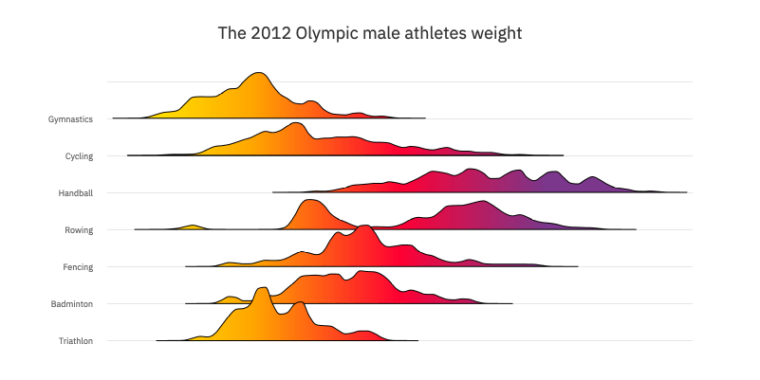# Density chartIn this tutorial, we will show you how to create multiple density plots, also know as ridgeline plot, using the Area Spline chart type. As you will see from the demo below, out result doesn’t look much like our ‘default’ Area Spline chart demo, but here is where Highcharts really excels: While 99% of use cases can be satisfied with running our charts with all default settings, a lot of fun can be had by examining the API as well as considering what you want your data story to be and how to best pre-process your data to that end.

In this tutorial, we will do just that, continuing our exploration of the super-useful Kernel Density Estimation. We used a Gaussian kernel function in previous tutorials to create a single density chart and violin chart types. And you might ask: “wait a second, isn’t a violin chart and the density chart, in essence, the same chart?” And you would be right. A density chart is basically a violin chart split down the middle, where only one of the halves is used. Even at half size, the density chart doesn’t lose any information, as the left and right side of the violin is just a mirror image of each other.

So, when would you use a violin or density chart? Both chart types are used to visualize a data distribution and its probability density. The shape of both charts gets tinner in lower density values and thicker in higher density values. While they can both be used to show the same data, you might consider going with a density chart for compact and straightforward data distribution visualization. Use the violin chart to display an attractive density distribution with descriptive statistical coefficients.

Good? Let’s return to our main subject and harness the density type chart’s power in comparing multiple series.

The demo below displays the weight density of the 2012 Olympic male athletes for six disciplines:

The chart is easy to read thanks to the density shape and the linear gradient. The audience can see and analyze the data spread using the chart shape, and compare the weight using the color gradient.
The code behind the chart is also easy to understand. The main function that processes the density data `processDensity()` (check the GitHub link) is inspired by the article Kernel Density Estimation. The function gets four main parameters and returns three arrays:

```function processDensity(step, precision, densityWidth, ...args) {
…
return {
xiData,
results,
stat
};
}```

Here is the description of the function’s parameters:

• `step` is the minimum data set unit. The step is used to sample the data set and create the KDE.
• `precision` is used to refine the density plot at the extremities, and in the thin spots, the smallest this parameter is the more points you get on the extremities and the thin spots on the chart.
• `densityWidth` is used to widen the density. This parameter should be equal to 1 to reflect the result of the KDE values. Nevertheless, for visibility purposes, you are free to change the `densityWidth` to get a wider and visible shape.
• `args` is one or many arrays that represent the data set. In our case, args is four arrays of weight athletes, one array for each discipline.

The code below is an example of how to use the function:

```let step = 1,
precision = 0.00000000001,
width = 15;
let data = processDensity(
step,
precision,
width,
dataArray, //triathlon,
dataArray, //fencing,
dataArray, //rowing,
dataArray, //handball,
dataArray, //cycling,
dataArray //gymnastics
);```

Here is the description of the returned arrays:

• `xiData` is the xAxis data generated using the step and the range of the athletes’ weights data.
• `results` include all the density charts data.
• `stat` is the array with all the descriptive statistical coefficients.

Once the density data, for each series, is generated thanks to the `processDensity()` function, the next step is to render the data.

To visualize the data an `areasplinerange` type chart is used, as the `processDensity()` returns a data array results according to the `areasplinerange` format.

```  Highcharts.chart("container", {
chart: {
type: "areasplinerange",
…```

To create the color gradient effect we use the `linearGradient` option:

```events: {
render() {
if (!redrawing) {
redrawing = true;

this.series.forEach((s) => {
s.update({
fillColor: {
stops: [
[0, Highcharts.color("yellow").setOpacity(0).get("rgba")],
[0.25, "orange"],
[0.5, "red"],
[0.6, "purple"]
]
}
});
});
redrawing = false;
}
}
}```

Be sure to use the `linearGradient` in an `event` under the `chart` feature; otherwise, the gradient effect will not be the same in different screen sizes.

By now, you are well equipped to create a compelling density chart with Highcharts. Feel free to try this code to create an interactive density chart or to come up with your own algorithm then share it with the community in the comment section below.